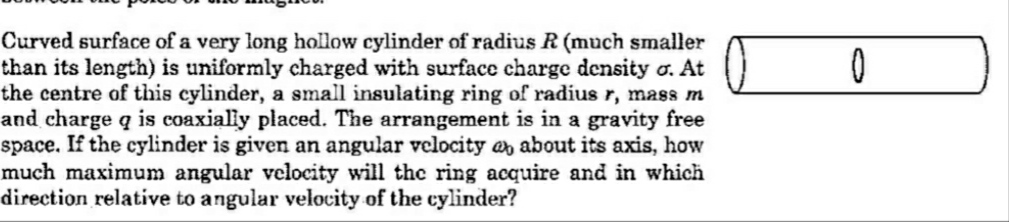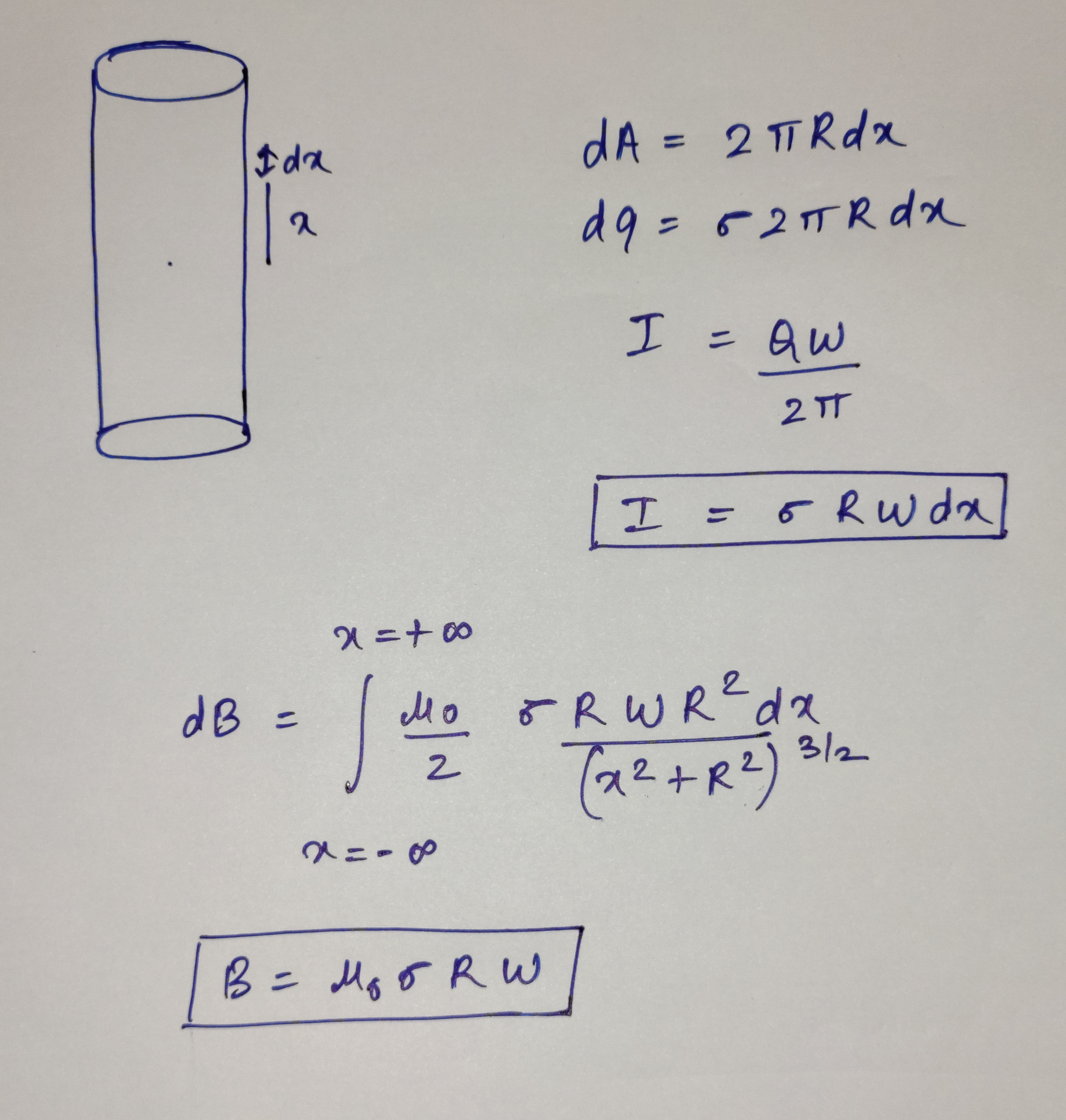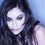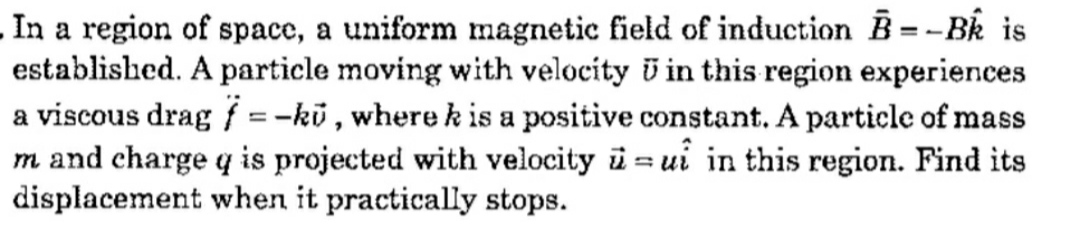# Stucking in a Magnetism problem

Here is the problem taken from Pathfinder Physics BookHere is my attemptI have calculated magnetic field at that ring, now how to proceed?
Should I use the concept generating electric field due to changing of magnetic field.Note by Lil Doug
2 weeks, 6 days ago

This discussion board is a place to discuss our Daily Challenges and the math and science related to those challenges. Explanations are more than just a solution — they should explain the steps and thinking strategies that you used to obtain the solution. Comments should further the discussion of math and science.

When posting on Brilliant:

• Use the emojis to react to an explanation, whether you're congratulating a job well done , or just really confused .
• Ask specific questions about the challenge or the steps in somebody's explanation. Well-posed questions can add a lot to the discussion, but posting "I don't understand!" doesn't help anyone.
• Try to contribute something new to the discussion, whether it is an extension, generalization or other idea related to the challenge.

MarkdownAppears as
*italics* or _italics_ italics
**bold** or __bold__ bold
- bulleted- list
• bulleted
• list
1. numbered2. list
1. numbered
2. list
Note: you must add a full line of space before and after lists for them to show up correctly
paragraph 1paragraph 2

paragraph 1

paragraph 2

[example link](https://brilliant.org)example link
> This is a quote
This is a quote
    # I indented these lines
# 4 spaces, and now they show
# up as a code block.

print "hello world"
# I indented these lines
# 4 spaces, and now they show
# up as a code block.

print "hello world"
MathAppears as
Remember to wrap math in $$ ... $$ or $ ... $ to ensure proper formatting.
2 \times 3 $2 \times 3$
2^{34} $2^{34}$
a_{i-1} $a_{i-1}$
\frac{2}{3} $\frac{2}{3}$
\sqrt{2} $\sqrt{2}$
\sum_{i=1}^3 $\sum_{i=1}^3$
\sin \theta $\sin \theta$
\boxed{123} $\boxed{123}$

Sort by:

@Steven Chase @Karan Chatrath

- 2 weeks, 6 days ago

WTF, why I am not able to mention names? This bug should be fixed.

- 2 weeks, 6 days ago

Yes, I think you need to apply the concept of an induced electric field. It is the resulting electric field that will cause the ring to rotate.

- 2 weeks, 6 days ago

@Karan Chatrath ....

- 2 weeks, 6 days ago

@Karan Chatrath sir the magnetic field is getting constant after $dt$ time?
I don't think it will help?
What do you think , this is the main concept $\vec{E}=-\frac{R}{2}\frac{d\vec{B}}{dt}$
The magnetic field which I have obtained above is $B=\mu_{0} \sigma R \omega$
And it is constant

- 2 weeks, 6 days ago

Okay, I will try to work it out by myself in some time. Let me give this a thought.

- 2 weeks, 5 days ago

@Karan Chatrath I have a special problem in this types of problems.Jaise maine is type ke question ka solution dekh lia maan lo kahi se (but iska solution internet pe available nhi hai) , to mujhe ye samajh aa jaayega, but jab mai khud se solve karne jaauga to nhi hoga.

- 2 weeks, 5 days ago

Startt by calculating all forces on the particle and applying Newton's second law

- 2 weeks, 5 days ago

@Karan Chatrath ha ha ha nhi ho rha sir, difficulty aa rhi?
The problem is that magnetics field se particle rotate karega, phir vaha pe drag lagega, bahut confusion ho rha,
Bas start kaise karu ye bata dijiye please

- 2 weeks, 5 days ago

Newton's second law:

$m \vec{a} = q(\vec{v} \times \vec{B}) -k \vec{v}$

You will get two equations for accelerations along X and Y. Solve those differential equations. The equations will be linear so you can solve for an exact solution.

- 2 weeks, 5 days ago

@Karan Chatrath $\Huge Thank$ $\Huge You$ $\Huge SO$ $\Huge MUCH$

- 2 weeks, 5 days ago